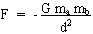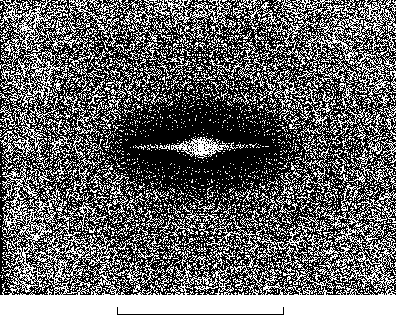Repulsive dark matter (p1)

Repulsive dark matter.

Jean-Pierre Petit and P.Midy
Observatory of Marseille,France

Abstract :

We explore the phenomenological aspects of a two population system whose dynamics implies both attractive and repelling forces. A new geometrical structure, with two folds, associated to two field equations, coupled through the gravitational field, allows to bypass the stumbling block of the "negative masses" and makes such a conceivable system, where the energies of all the particles are positive. We show that in these conditions, the matter in the second population is geometrically non-observable and therefore gets the status of repulsive dark matter. Galaxies would be housed in cavities of a homogenous distribution of repulsive dark matter. This generates a confinment effect with realistic rotation curve. We show that the negative gravitational lensing, associated with repulsive dark matter would explain the strong observed effects, which would be an alternative to the "classical" dark matter model. As derived from this new cosmological model, the age of the Universe becomes 15.7 billions years, due to the interaction of the two matters.

1) Introduction.

...Nowadays trying to account for astronomical observations on the basis of observable matter only is no longer possible. That is for dark matter concept has become increasingly widespread. Various hypothesis have been proposed about the nature of this unobserved component of the Universe, which should contribute to the formation of the gravitational field and thus lead to the missing mass effect in galaxies and to gravitational lensing. Machos have turned out to be disappointing. Some people resort to particles whose physical existence is speculative, such as massive neutrinos. In so far no formulation seems to have prevailed and many hypothesis remain possible about this dark matter. In this paper we propose to investigate the consequences of a gravitational interaction between our matter (mass m) and particular dark matter, made of masses m*, such that :

- m and m attract each other according to the Newton law

- m* and m* attract each other according to the Newton law

- m and m* repel each other according to a Newton-like law

We shall call m* "repulsive dark matter".

This could be summed up simply, considering that in the following expression :
(1)where the masses ma and mb can be positive or negative. Physicists could argue immediately, saying that particles with negative masses also have negative energies, which lacks physical meaning. In the section 3 of the article we shall propose a new geometrical context which makes possible the interaction of two populations, with masses m and m*, both positive, whose energies mc2 and m*c2 are positive, in such a way that the forces fit with the former scheme. The fact that the two sub-systems may interact only through gravitation will be geometrically justified.

.

2) Repulsive dark matter confining galaxies.

...I has been known for long that the gravitational field due to a matter distribution inferred from observation would not be able to balance the centrifugal forces in galaxies. The missin mass is about three to five times the observed one. Moreover, the rotation curves of galaxies show a characteristic step (peripheral excess velocities) which cannot be accounted for starting from the observed distribution of matter. So people attempts both to prevent explosion of galaxies and to reproduce this aspect of rotation curves using ad hoc, artificially introduced distributions of dark matter. Let us now turn to the proposed model of ordinary (observed) matter and unobserved repulsive dark matter, and see whether this model can ensure the confinment of galaxies. At first, we consider a galaxy where matter is distributed according to the model of Myamoto and Nagai  :
(2)...This axisymmetrical matter distribution is supposed to be located in a hole of a uniform repulsive dark matter distribution (Fig.1, in which ordinary matter is distributed according to a = 5 ; b = 1 in (2).Fig.1 : The galaxy surrounded by repulsive dark matter. An axisymmetrical system.

...We arrange repulsive dark matter around it, with an ad hoc density gradient, shaped on empirical grounds. This mass distribution can be described through a superposition of thick ellipsoids, charged by matter density ri (which could be positive or negative), i being the index of the massive ellipsoid, with horizontal axis ai and vertical axis bi. The field, inside and outside such bodies, is given by quite simple analytical formulas ( and ). Given a set of such massive ellipsoids it becomes possible to compute the 3d field. On figure 1 we have figured the mass-density r* of repulsive dark matter by the variation of the density of white points in space. This does no result from numerical simulation, performed with mass points, as the image might suggest. The mass distribution was described by a set of massive ellipsoïds, with various parameters (lenghts of axis, mass-density).

...Figure 2 shows the corresponding chosen axisymmetric repulsive dark matter distribution. Figure 3 represents the gravitational field, due to this repulsive dark matter, computed by the method described above. The two scales of figures 1 and (2-3) are different, the last ones being a zoom. The scale correspondance is indicated. As we can see, the repulsive dark matter distribution produces a confinment effect on the galaxy, both in the r and z-directions. Figure 3 shows the corresponding rotation velocity for repulsive dark matter alone. We see that such a repulsive dark matter distribution allows large peripheric velocities.Fig. 2 : The chosen repulsive dark matter distribution : a set of thick, concentric flat ellispsoids with density r*(r) d being the diameter of the ellipsoid.